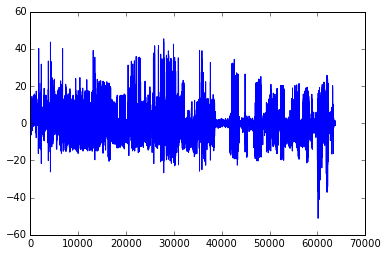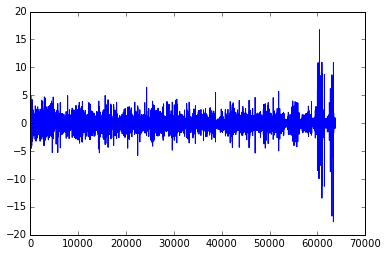## Large-scale bundle adjustment in scipy¶

A bundle adjusmtent problem arises in 3-D reconstruction and it can be formulated as follows (taken from https://en.wikipedia.org/wiki/Bundle_adjustment):

Given a set of images depicting a number of 3D points from different viewpoints, bundle adjustment can be defined as the problem of simultaneously refining the 3D coordinates describing the scene geometry as well as the parameters of the relative motion and the optical characteristics of the camera(s) employed to acquire the images, according to an optimality criterion involving the corresponding image projections of all points.

More precisely. We have a set of points in real world defined by their coordinates $(X, Y, Z)$ in some apriori chosen "world coordinate frame". We photograph these points by different cameras, which are characterized by their orientation and translation relative to the world coordinate frame and also by focal length and two radial distortion parameters (9 parameters in total). Then we precicely measure 2-D coordinates $(x, y)$ of the points projected by the cameras on images. Our task is to refine 3-D coordinates of original points as well as camera parameters, by minimizing the sum of squares of reprojecting errors.

Let $\pmb{P} = (X, Y, Z)^T$ - a radius-vector of a point, $\pmb{R}$ - a rotation matrix of a camera, $\pmb{t}$ - a translation vector of a camera, $f$ - its focal distance, $k_1, k_2$ - its distortion parameters. Then the reprojecting is done as follows:

\begin{align} \pmb{Q} = \pmb{R} \pmb{P} + \pmb{t} \\ \pmb{q} = -\begin{pmatrix} Q_x / Q_z \\ Q_y / Q_z \end{pmatrix} \\ \pmb{p} = f (1 + k_1 \lVert \pmb{q} \rVert^2 + k_2 \lVert \pmb{q} \rVert^4) \pmb{q} \end{align}

The resulting vector $\pmb{p}=(x, y)^T$ contains image coordinates of the original point. This model is called "pinhole camera model", a very good notes about this subject I found here http://www.comp.nus.edu.sg/~cs4243/lecture/camera.pdf

Now let's start solving some real bundle adjusment problem. We'll take a problem from http://grail.cs.washington.edu/projects/bal/.

In :
import urllib
import bz2
import os
import numpy as np


In :
BASE_URL = "http://grail.cs.washington.edu/projects/bal/data/ladybug/"
FILE_NAME = "problem-49-7776-pre.txt.bz2"
URL = BASE_URL + FILE_NAME

In :
if not os.path.isfile(FILE_NAME):
urllib.request.urlretrieve(URL, FILE_NAME)


Now read the data from the file:

In :
def read_bal_data(file_name):
with bz2.open(file_name, "rt") as file:
n_cameras, n_points, n_observations = map(

camera_indices = np.empty(n_observations, dtype=int)
point_indices = np.empty(n_observations, dtype=int)
points_2d = np.empty((n_observations, 2))

for i in range(n_observations):
camera_index, point_index, x, y = file.readline().split()
camera_indices[i] = int(camera_index)
point_indices[i] = int(point_index)
points_2d[i] = [float(x), float(y)]

camera_params = np.empty(n_cameras * 9)
for i in range(n_cameras * 9):
camera_params = camera_params.reshape((n_cameras, -1))

points_3d = np.empty(n_points * 3)
for i in range(n_points * 3):
points_3d = points_3d.reshape((n_points, -1))

return camera_params, points_3d, camera_indices, point_indices, points_2d

In :
camera_params, points_3d, camera_indices, point_indices, points_2d = read_bal_data(FILE_NAME)


Here we have numpy arrays:

1. camera_params with shape (n_cameras, 9) contains initial estimates of parameters for all cameras. First 3 components in each row form a rotation vector (https://en.wikipedia.org/wiki/Rodrigues%27_rotation_formula), next 3 components form a translation vector, then a focal distance and two distortion parameters.
2. points_3d with shape (n_points, 3) contains initial estimates of point coordinates in the world frame.
3. camera_ind with shape (n_observations,) contains indices of cameras (from 0 to n_cameras - 1) involved in each observation.
4. point_ind with shape (n_observations,) contatins indices of points (from 0 to n_points - 1) involved in each observation.
5. points_2d with shape (n_observations, 2) contains measured 2-D coordinates of points projected on images in each observations.

And the numbers are:

In :
n_cameras = camera_params.shape
n_points = points_3d.shape

n = 9 * n_cameras + 3 * n_points
m = 2 * points_2d.shape

print("n_cameras: {}".format(n_cameras))
print("n_points: {}".format(n_points))
print("Total number of parameters: {}".format(n))
print("Total number of residuals: {}".format(m))

n_cameras: 49
n_points: 7776
Total number of parameters: 23769
Total number of residuals: 63686


We chose a relatively small problem to reduce computation time, but scipy's algorithm is capable of solving much larger problems, although required time will grow proportionally.

Now define the function which returns a vector of residuals. We use numpy vectorized computations:

In :
def rotate(points, rot_vecs):
"""Rotate points by given rotation vectors.

Rodrigues' rotation formula is used.
"""
theta = np.linalg.norm(rot_vecs, axis=1)[:, np.newaxis]
with np.errstate(invalid='ignore'):
v = rot_vecs / theta
v = np.nan_to_num(v)
dot = np.sum(points * v, axis=1)[:, np.newaxis]
cos_theta = np.cos(theta)
sin_theta = np.sin(theta)

return cos_theta * points + sin_theta * np.cross(v, points) + dot * (1 - cos_theta) * v

In :
def project(points, camera_params):
"""Convert 3-D points to 2-D by projecting onto images."""
points_proj = rotate(points, camera_params[:, :3])
points_proj += camera_params[:, 3:6]
points_proj = -points_proj[:, :2] / points_proj[:, 2, np.newaxis]
f = camera_params[:, 6]
k1 = camera_params[:, 7]
k2 = camera_params[:, 8]
n = np.sum(points_proj**2, axis=1)
r = 1 + k1 * n + k2 * n**2
points_proj *= (r * f)[:, np.newaxis]
return points_proj

In :
def fun(params, n_cameras, n_points, camera_indices, point_indices, points_2d):
"""Function which computes residuals.

params contains camera parameters and 3-D coordinates.
"""
camera_params = params[:n_cameras * 9].reshape((n_cameras, 9))
points_3d = params[n_cameras * 9:].reshape((n_points, 3))
points_proj = project(points_3d[point_indices], camera_params[camera_indices])
return (points_proj - points_2d).ravel()


You can see that computing Jacobian of fun is cumbersome, thus we will rely on the finite difference approximation. To make this process time feasible we provide Jacobian sparsity structure (i. e. mark elements which are known to be non-zero):

In :
from scipy.sparse import lil_matrix

In :
def bundle_adjustment_sparsity(n_cameras, n_points, camera_indices, point_indices):
m = camera_indices.size * 2
n = n_cameras * 9 + n_points * 3
A = lil_matrix((m, n), dtype=int)

i = np.arange(camera_indices.size)
for s in range(9):
A[2 * i, camera_indices * 9 + s] = 1
A[2 * i + 1, camera_indices * 9 + s] = 1

for s in range(3):
A[2 * i, n_cameras * 9 + point_indices * 3 + s] = 1
A[2 * i + 1, n_cameras * 9 + point_indices * 3 + s] = 1

return A


Now we are ready to run optimization. Let's visualize residuals evaluated with the initial parameters.

In :
%matplotlib inline
import matplotlib.pyplot as plt

In :
x0 = np.hstack((camera_params.ravel(), points_3d.ravel()))

In :
f0 = fun(x0, n_cameras, n_points, camera_indices, point_indices, points_2d)

In :
plt.plot(f0)

Out:
[<matplotlib.lines.Line2D at 0x107584828>]In :
A = bundle_adjustment_sparsity(n_cameras, n_points, camera_indices, point_indices)

In :
import time
from scipy.optimize import least_squares

In :
t0 = time.time()
res = least_squares(fun, x0, jac_sparsity=A, verbose=2, scaling='jac', ftol=1e-4,
args=(n_cameras, n_points, camera_indices, point_indices, points_2d))
t1 = time.time()

   Iteration     Total nfev        Cost      Cost reduction    Step norm     Optimality
0              1         8.5091e+05                                    8.57e+06
1              3         5.0985e+04      8.00e+05       1.46e+02       1.15e+06
2              4         1.6077e+04      3.49e+04       2.59e+01       2.43e+05
3              5         1.4163e+04      1.91e+03       2.86e+02       1.21e+05
4              7         1.3695e+04      4.67e+02       1.32e+02       2.51e+04
5              8         1.3481e+04      2.14e+02       2.24e+02       1.54e+04
6              9         1.3436e+04      4.55e+01       3.17e+02       2.72e+04
7             10         1.3422e+04      1.36e+01       6.82e+01       2.19e+03
8             11         1.3418e+04      3.67e+00       1.25e+02       7.77e+03
9             12         1.3414e+04      4.11e+00       2.64e+01       6.29e+02
10             13         1.3412e+04      1.88e+00       7.44e+01       2.59e+03
11             14         1.3410e+04      2.07e+00       1.77e+01       4.94e+02
12             15         1.3409e+04      1.04e+00       3.98e+01       1.33e+03
ftol termination condition is satisfied.
Function evaluations: 15, initial cost: 8.5091e+05, final cost 1.3409e+04, first-order optimality 1.33e+03.

In :
print("Optimization took {} seconds".format(int(t1 - t0)))

Optimization took 45 seconds


Setting scaling='jac' was done to automatically scale the variables and equalize their influence on the cost function (clearly the camera parameters and coordinates of the points are very different entities). This option turned out to be crucial for successfull bundle adjustment.

Now let's plot residuals at the found solution:

In :
plt.plot(res.fun)

Out:
[<matplotlib.lines.Line2D at 0x10b47a2b0>]We see much better picture of residuals now, with the mean being very close to zero. There are some spikes left. It can be explained by outliers in the data, or, possibly, the algorithm found a local minimum (very good one though) or didn't converged enough. Note that the algorithm worked with Jacobian finite difference aproximate, which can potentially block the progress near the minimum because of insufficient accuracy (but again, computing exact Jacobian for this problem is quite difficult).